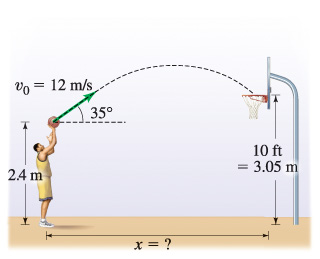# Problem: A basketball is shot from an initial height of 2.4 m with an initial speed v0 =12 m/s directed at an angle 0 = 35 above the horizontal.How far from the basket was the player if he made a basket?At what angle to the horizontal did the ball enter the basket?

###### FREE Expert Solution
94% (277 ratings)
###### Problem Details

A basketball is shot from an initial height of 2.4 m with an initial speed v0 =12 m/s directed at an angle 0 = 35 above the horizontal.